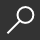## Average Time Complexity of Decision Trees by Igor Chikalov

Overview -

Decision tree is a widely used form of representing algorithms and knowledge. Compact data models

and fast algorithms require optimization of tree complexity. This book is a research monograph on

average time complexity of decision trees. It generalizes several known results and considers a number of new problems.

The book contains exact and approximate algorithms for decision tree optimization, and bounds on minimum average time

complexity of decision trees. Methods of combinatorics, probability theory and complexity theory are used in the proofs as

well as concepts from various branches of discrete mathematics and computer science.The considered applications include

the study of average depth of decision trees for Boolean functions from closed classes, the comparison of results of the performance

of greedy heuristics for average depth minimization with optimal decision trees constructed by dynamic programming algorithm,

and optimization of decision trees for the corner point recognition problem from computer vision.

The book can be interesting for researchers working on time complexity of algorithms and specialistsin test theory, rough set theory, logical analysis of data and machine learning.

local_shippingFor DeliveryIn Stock.
This item is Non-Returnable.
FREE Shipping for Club Members helpNew & Used Marketplace 5 copies from \$131.35

## More About Average Time Complexity of Decision Trees by Igor Chikalov

### Overview

Decision tree is a widely used form of representing algorithms and knowledge. Compact data models

and fast algorithms require optimization of tree complexity. This book is a research monograph on

average time complexity of decision trees. It generalizes several known results and considers a number of new problems.

The book contains exact and approximate algorithms for decision tree optimization, and bounds on minimum average time

complexity of decision trees. Methods of combinatorics, probability theory and complexity theory are used in the proofs as

well as concepts from various branches of discrete mathematics and computer science.The considered applications include

the study of average depth of decision trees for Boolean functions from closed classes, the comparison of results of the performance

of greedy heuristics for average depth minimization with optimal decision trees constructed by dynamic programming algorithm,

and optimization of decision trees for the corner point recognition problem from computer vision.

The book can be interesting for researchers working on time complexity of algorithms and specialistsin test theory, rough set theory, logical analysis of data and machine learning.

This item is Non-Returnable.

### Details

• ISBN-13: 9783642270161
• ISBN-10: 3642270166
• Publisher: Springer
• Publish Date: November 2013
• Page Count: 104
• Dimensions: 9.21 x 6.14 x 0.24 inches
• Shipping Weight: 0.38 pounds

Series: Intelligent Systems Reference Library #21Related Categories